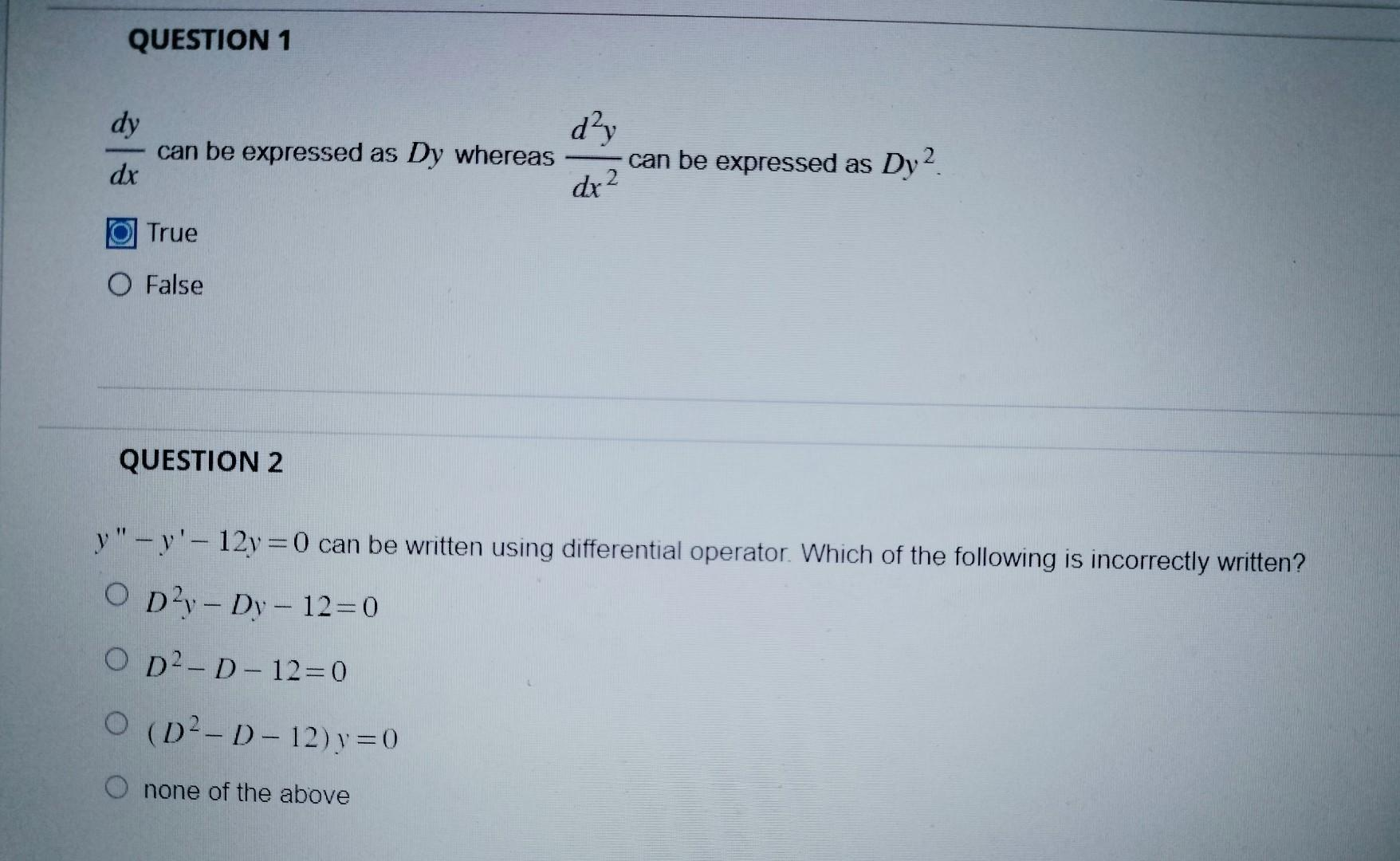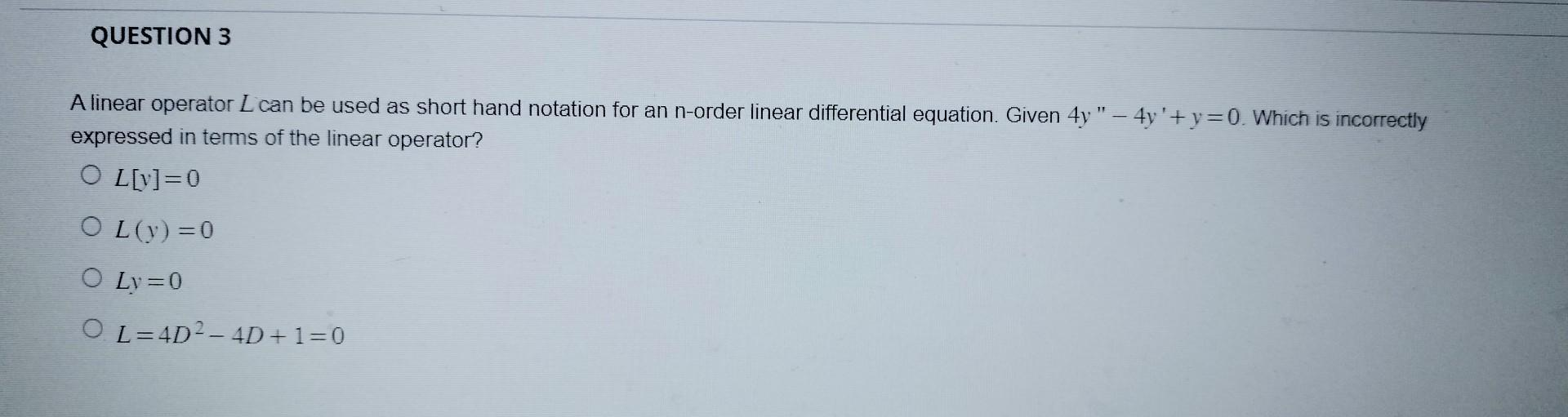# Question     pls help me with this three questions thank you. I will upvote for it $$\frac{d y}{d x}$$ can be expressed as $$D y$$ whereas $$\frac{d^{2} y}{d x^{2}}$$ can be expressed as $$D y^{2}$$ True False QUESTION 2 $$y^{\prime \prime}-y^{\prime}-12 y=0$$ can be written using differential operator. Which of the following is incorrectly written? $\begin{array}{l} D^{2} y-D y-12=0 \\ D^{2}-D-12=0 \\ \left(D^{2}-D-12\right) y=0 \end{array}$ none of the above A linear operator $$L$$ can be used as short hand notation for an n-order linear differential equation. Given $$4 y^{\prime \prime}-4 y^{\prime}+y=0$$. Which is incorrectly expressed in terms of the linear operator? $\begin{array}{l} L[y]=0 \\ L(y)=0 \\ L y=0 \\ L=4 D^{2}-4 D+1=0 \end{array}$pls help me with this three questions thank you. I will upvote for it

Transcribed Image Text: $$\frac{d y}{d x}$$ can be expressed as $$D y$$ whereas $$\frac{d^{2} y}{d x^{2}}$$ can be expressed as $$D y^{2}$$ True False QUESTION 2 $$y^{\prime \prime}-y^{\prime}-12 y=0$$ can be written using differential operator. Which of the following is incorrectly written? $\begin{array}{l} D^{2} y-D y-12=0 \\ D^{2}-D-12=0 \\ \left(D^{2}-D-12\right) y=0 \end{array}$ none of the above A linear operator $$L$$ can be used as short hand notation for an n-order linear differential equation. Given $$4 y^{\prime \prime}-4 y^{\prime}+y=0$$. Which is incorrectly expressed in terms of the linear operator? $\begin{array}{l} L[y]=0 \\ L(y)=0 \\ L y=0 \\ L=4 D^{2}-4 D+1=0 \end{array}$
More
Transcribed Image Text: $$\frac{d y}{d x}$$ can be expressed as $$D y$$ whereas $$\frac{d^{2} y}{d x^{2}}$$ can be expressed as $$D y^{2}$$ True False QUESTION 2 $$y^{\prime \prime}-y^{\prime}-12 y=0$$ can be written using differential operator. Which of the following is incorrectly written? $\begin{array}{l} D^{2} y-D y-12=0 \\ D^{2}-D-12=0 \\ \left(D^{2}-D-12\right) y=0 \end{array}$ none of the above A linear operator $$L$$ can be used as short hand notation for an n-order linear differential equation. Given $$4 y^{\prime \prime}-4 y^{\prime}+y=0$$. Which is incorrectly expressed in terms of the linear operator? $\begin{array}{l} L[y]=0 \\ L(y)=0 \\ L y=0 \\ L=4 D^{2}-4 D+1=0 \end{array}$&#12304;General guidance&#12305;The answer provided below has been developed in a clear step by step manner.Step1/4Given:The given statement is $$\mathrm{\frac{{\left.{d}{y}\right.}}{{\left.{d}{x}\right.}}}$$ can be expressed as $$\mathrm{{D}{y}}$$ whereas $$\mathrm{\frac{{{d}^{{2}}{y}}}{{{\left.{d}{x}\right.}^{{2}}}}}$$ can be expressed as $$\mathrm{{D}{y}^{{2}}}$$.Objective:To establish if the assertion is accurate or not.Explanation:Please refer to solution in this step.Step2/4$$\mathrm{{1}{)}}$$ Consider the statement, $$\mathrm{\frac{{\left.{d}{y}\right.}}{{\left.{d}{x}\right.}}}$$ can be expressed as $$\mathrm{{D}{y}}$$ whereas $$\mathrm{\frac{{{d}^{{2}}{y}}}{{{\left.{d}{x}\right.}^{{2}}}}}$$ can be expressed as $$\mathrm{{D}{y}^{{2}}}$$.Since differential operator is $$\mathrm{{D}=\frac{{d}}{{\left.{d}{x}\right.}}}$$.Hence $$\mathrm{\frac{{\left.{d}{y}\right.}}{{\left.{d}{x}\right.}}}$$ can be expressed as $$\mathrm{{D}{y}}$$.But $$\mathrm{\frac{{{d}^{{2}}{y}}}{{{\left.{d}{x}\right.}^{{2}}}}}$$ cannot be expressed as $$\mathrm{{D}{y}^{{2}}}$$.It can be written as $$\mathrm{{D}^{{2}}{y}}$$. Here $$\mathrm{{D}{y}^{{2}}}$$ gives $$\mathrm{\frac{{{\left.{d}{y}\right.}^{{2}}}}{{{\left.{d}{x}\right.}}}}$$ hence it is not same as $$\mathrm{\frac{{{d}^{{2}}{y}}}{{{\left.{d}{x}\right.}^{{2}}}}}$$.Therefore the statement given is false.ExplanationEvery group of derivatives used on a function is known as a differential operator.Explanation:Please refer to solution in this step.Step3/4$$\mathrm{{2}{)}}$$ Consider the differential equation $$\mathrm{{y}{''}-{y}'-{12}{y}={0}}$$.To write it by using differential operator.Here assume $$\mathrm{{D}=\frac{{d}}{{\left.{d}{x}\right.}}}$$ and $$\mathrm{{D}^{{2}}=\frac{{d}^{{2}}}{{\left.{d}{x}\right.}^{{2}}}}$$.Consider the differential equation as $$\mathrm{{\left({f{{\left({D}\right)}}}\right)}{y}={0}}$$ with \( \mathrm{{D}^{{2}}-{ ... See the full answer